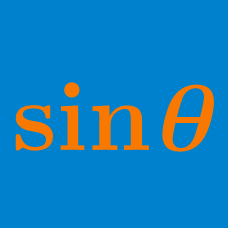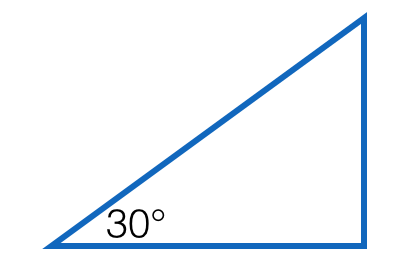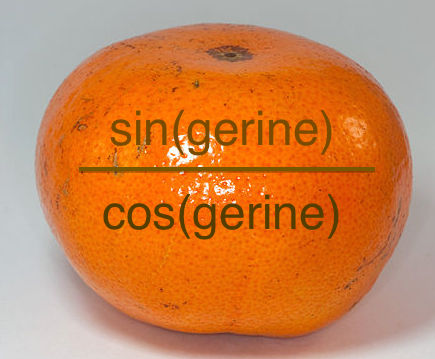Geometry

# Trigonometric Functions: Level 2 Challenges$\Large \sin \left(30^\circ\right) = \ ?$

$\cos(45^\circ) \times \cos(46^\circ) \times \cos(47^\circ) \times \ldots \times \cos(135^\circ ) = \ ?$

$\huge \frac{\hspace{2mm} \frac{\hspace{2mm} \frac{\hspace{2mm} \frac{\hspace{2mm} \frac{\sin x}{\cos x}\hspace{2mm} }{\tan x}\hspace{2mm} }{\cot x}\hspace{2mm} }{\sec x}\hspace{2mm} }{\csc x}$

Find the value of the above fraction tower if $$0^\circ < x < {90}^\circ$$.

True or False?

$\sin ^{-1} \left( \sin \theta \right) = \theta.$

The domain of $$\sin \theta$$ is all real numbers.Calculate

$\frac{ 9 \cot^2 60^\circ + \sec ^2 45 ^\circ - 4 \sin 90^\circ } { 3 \tan^2 30^\circ + \csc^2 45^\circ - \tan 45^\circ }.$

×